# F4 (mathematics)

In mathematics, F4 is the name of a Lie group and also its Lie algebra f4. It is one of the five exceptional simple Lie groups. F4 has rank 4 and dimension 52. The compact form is simply connected and its outer automorphism group is the trivial group. Its fundamental representation is 26-dimensional.

The compact real form of F4 is the isometry group of a 16-dimensional Riemannian manifold known as the octonionic projective plane OP2. This can be seen systematically using a construction known as the magic square, due to Hans Freudenthal and Jacques Tits.

There are 3 real forms: a compact one, a split one, and a third one. They are the isometry groups of the three real Albert algebras.

The F4 Lie algebra may be constructed by adding 16 generators transforming as a spinor to the 36-dimensional Lie algebra so(9), in analogy with the construction of E8.

In older books and papers, F4 is sometimes denoted by E4.

## Algebra

### Dynkin diagram

The Dynkin diagram for F4 is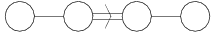.

### Weyl/Coxeter group

Its Weyl/Coxeter groupis the symmetry group of the 24-cell: it is a solvable group of order 1152. It has minimal faithful degreewhich is realized by the action on the 24-cell.

### Cartan matrix### F4 lattice

The F4 lattice is a four-dimensional body-centered cubic lattice (i.e. the union of two hypercubic lattices, each lying in the center of the other). They form a ring called the Hurwitz quaternion ring. The 24 Hurwitz quaternions of norm 1 form the vertices of a 24-cell centered at the origin.

### Roots of F4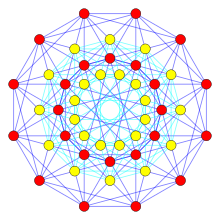The 24 vertices of 24-cell (red) and 24 vertices of its dual (yellow) represent the 48 root vectors of F4 in this Coxeter plane projection

The 48 root vectors of F4 can be found as the vertices of the 24-cell in two dual configurations:

24-cell vertices:• 24 roots by (±1,±1,0,0), permuting coordinate positions

Dual 24-cell vertices:• 8 roots by (±1, 0, 0, 0), permuting coordinate positions
• 16 roots by (±½, ±½, ±½, ±½).

#### Simple roots

One choice of simple roots for F4,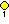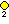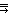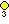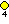, is given by the rows of the following matrix: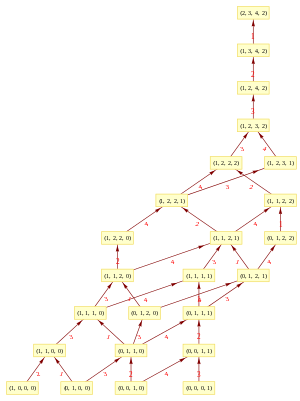Hasse diagram of F4 root poset with edge labels identifying added simple root position

### F4 polynomial invariant

Just as O(n) is the group of automorphisms which keep the quadratic polynomials x2 + y2 + ... invariant, F4 is the group of automorphisms of the following set of 3 polynomials in 27 variables. (The first can easily be substituted into other two making 26 variables).Where x, y, z are real valued and X, Y, Z are octonion valued. Another way of writing these invariants is as (combinations of) Tr(M), Tr(M2) and Tr(M3) of the hermitian octonion matrix:The set of polynomials defines a 24 dimensional compact surface.

## Representations

The characters of finite dimensional representations of the real and complex Lie algebras and Lie groups are all given by the Weyl character formula. The dimensions of the smallest irreducible representations are (sequence A121738 in the OEIS):

1, 26, 52, 273, 324, 1053 (twice), 1274, 2652, 4096, 8424, 10829, 12376, 16302, 17901, 19278, 19448, 29172, 34749, 76076, 81081, 100776, 106496, 107406, 119119, 160056 (twice), 184756, 205751, 212992, 226746, 340119, 342056, 379848, 412776, 420147, 627912

The 52-dimensional representation is the adjoint representation, and the 26-dimensional one is the trace-free part of the action of F4 on the exceptional Albert algebra of dimension 27.

There are two non-isomorphic irreducible representations of dimensions 1053, 160056, 4313088, etc. The fundamental representations are those with dimensions 52, 1274, 273, 26 (corresponding to the four nodes in the Dynkin diagram in the order such that the double arrow points from the second to the third).

1. Saunders, Neil (2014). "Minimal Faithful Permutation Degrees for Irreducible Coxeter Groups and Binary Polyhedral Groups". arXiv:0812.0182.